blog

# Probability Of Touch In Options: Is It Important?

#### Options Trading 101 - The Ultimate Beginners Guide To Options

As Seen On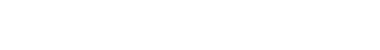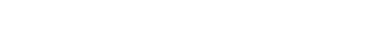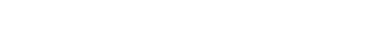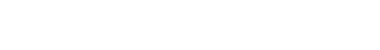by Gavin in BlogProbability of touch is an importance concept in the world of options. Lets take a look at what it is and how it works.

## Contents

Some trading platforms will give an investor the probability of a stock reaching a certain price at some point prior to the expiration of an option.

This is known as the probability of touch.

Let’s look at some examples.

## Example Using Cash-Secured Put

On November 25, 2020 at end of market day, CAT (Caterpillar) is trading at \$174.63.

An investor is willing (and has the capital in the account) to buy 100 shares of CAT if price drops back down to \$160 near the 50-day simple moving average.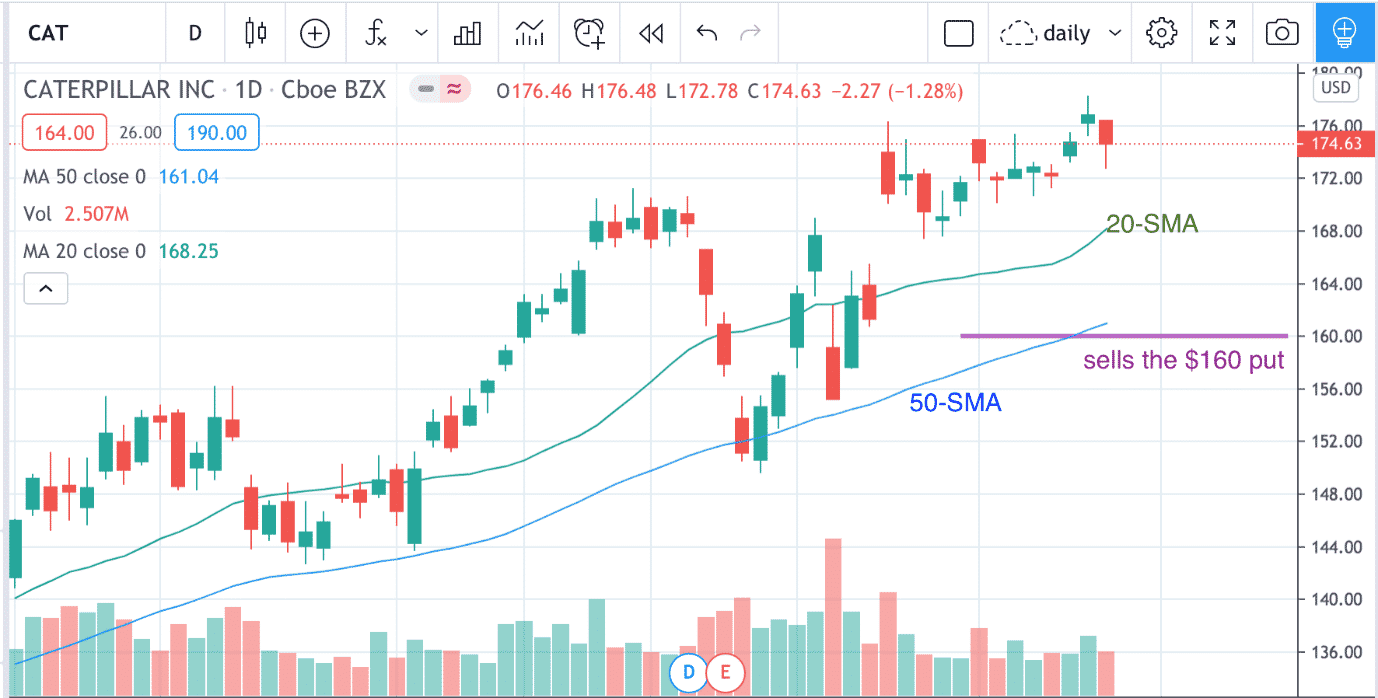The investor sells one put option on CAT at strike price of \$160 with expiration in 23 days on December 18.

With the ThinkOrSwim platform configured to show Delta and Probability of Touch, we see that the \$160 strike corresponds to the 14 delta.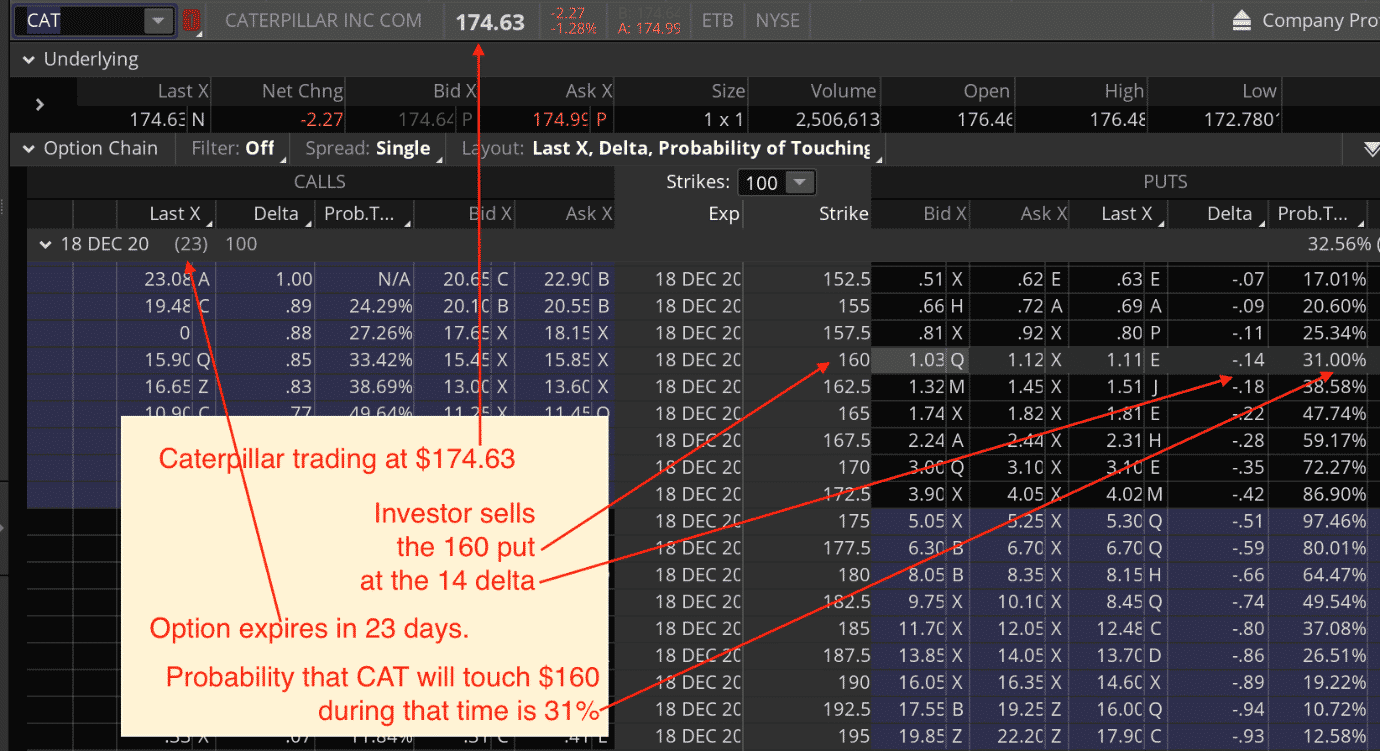The delta can be thought of as an estimate of how likely the option will be in-the-money at expiration.

In this case, there is a 14% chance that the \$160 put option will be in-the-money at expiration on December 18.

We also see that there is a 31% probability of touch.

In other words, the price of CAT has a 31% chance of touching \$160 at some time prior to expiration.

It makes intuitive sense that the probability of temporarily touching the price is greater than the probability of price ending up below the price expiration.

Roughly, it is about to twice as likely.

Because 2 x 14% is 28% which is close to 31%.

In a trade like this, whenever price reaches the short strike, the investor is on the brink of a loss.

That is why adjusting is such an important skill.

Nevertheless, if the investor does find the strike price being tested, then about half the time, a loss does not occur (the price reverses in investor’s favor) and about half the time, the loss does in fact occur — assuming that the option is held to expiration.

## Example Using Long Call

Suppose another investor is bullish on the overall market and decides to buy one call contract on the SPY with 50 days to expiration.

Date: November 25, 2020

Underlying Price: \$326.66

Buy one SPY Jan 15, 2021 – \$374 call @ \$4.00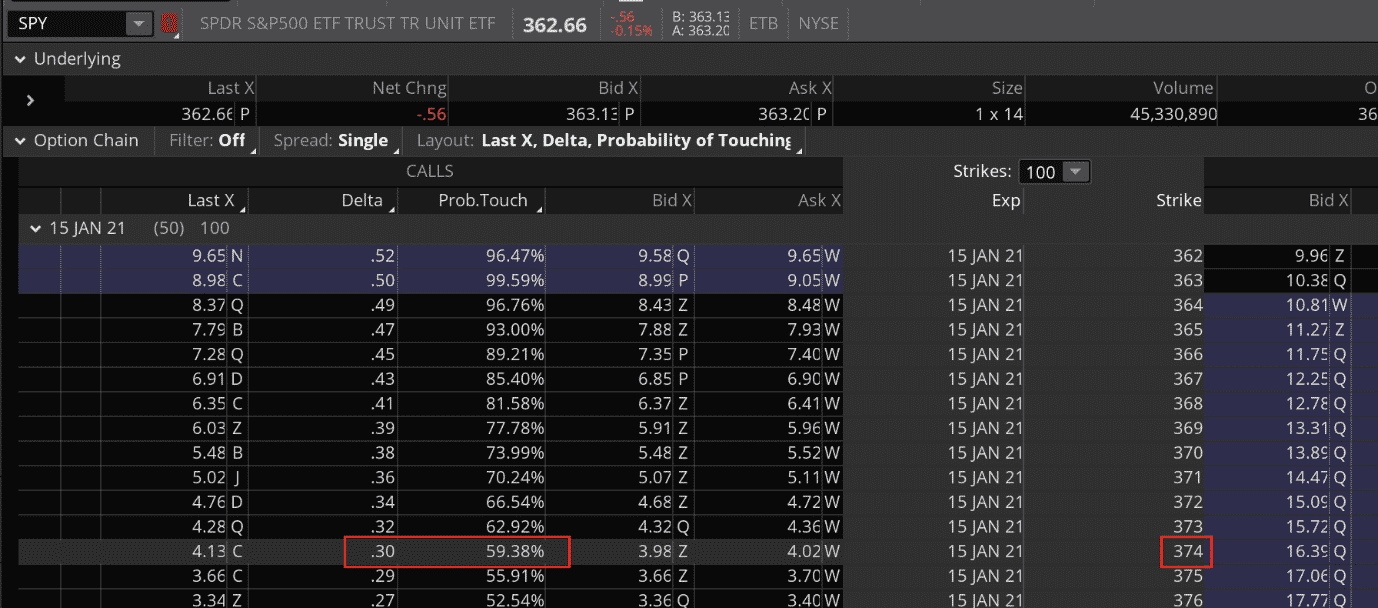The strike price of \$374 corresponds to 30 delta, meaning there is a roughly 30% chance that SPY will be above \$374 at expiration.

We also see that the probability of touch is 59% — about twice as likely to touch \$374 than ending below \$374 at expiration.

When investors talk about the probability of touch, it is almost always on out-of-the-money options.

## General Rule of Thumb

Looking at the probability of touch on the RUT Russell 2000 Index, we see again that the probability of touch is roughly twice the delta.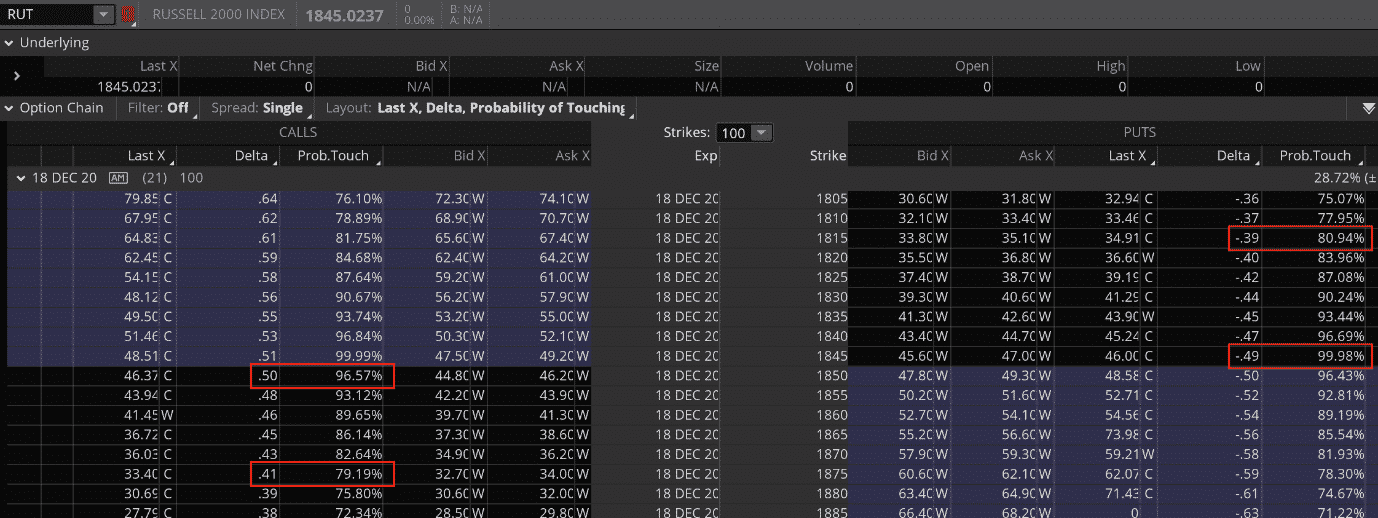We also see that the probability of touch is higher for strikes closer to the money, which makes sense.

From these three examples, we have a generalized rule-of-thumb that the probability of touch for out-of-the-money options is roughly twice the delta.

It works for both the call side and the put side.

But sometimes there is a probability skew between the calls and puts as we shall see.

This rule is only a rough rule-of-thumb, certain volatile stocks may not cater to this rule as much.

For example, take a look at TSLA.

When compared with CAT, it has a much higher ATR (Average True Range) relative to price.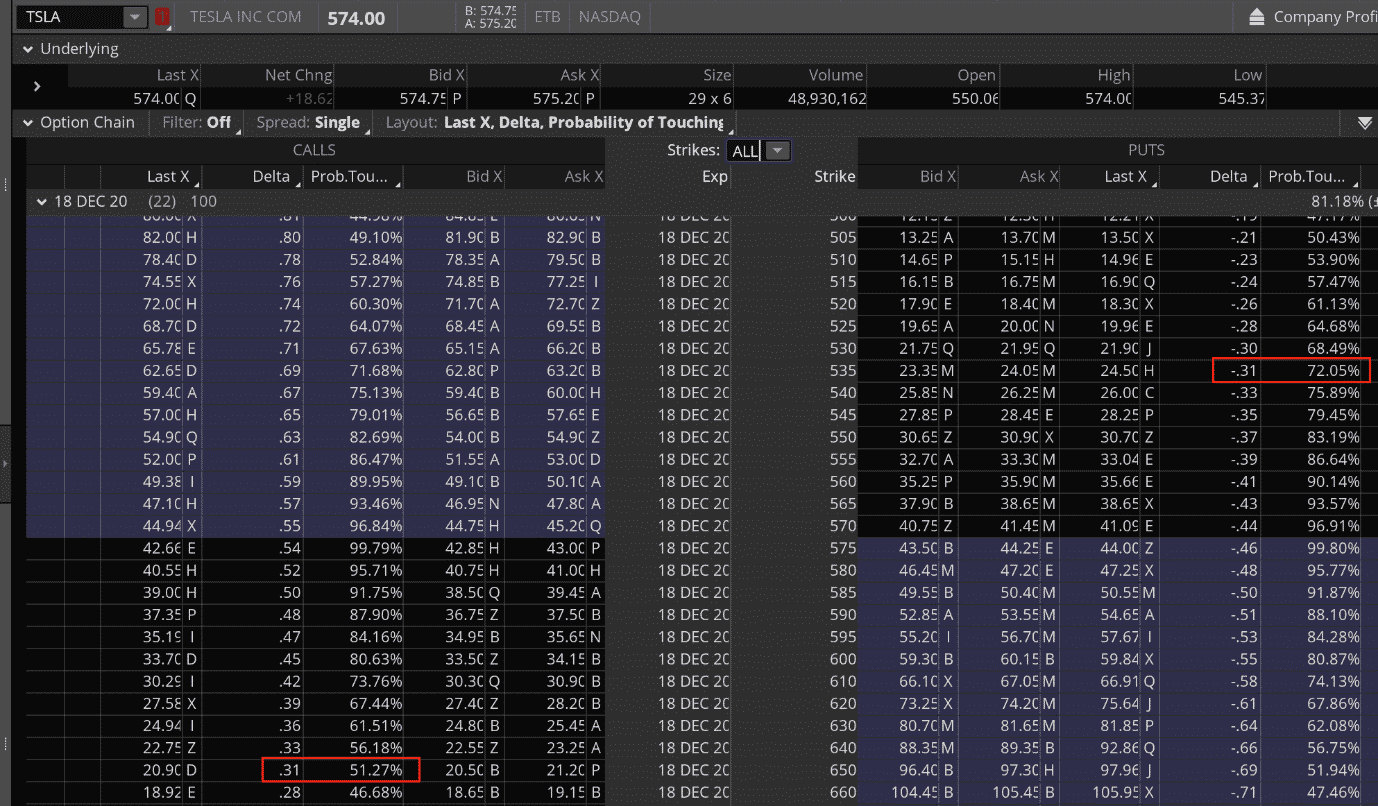The probability of touch of the 31 delta put is computed by ThinkOrSwim as 72% — quite a bit more than twice the delta.

The probability of touch of the 31 delta call is shown as 51% — quite a bit less than twice the delta.

This suggests that for TSLA at this expiration cycle, the probability of touch on puts is higher than the probability of touch on calls (for the same delta).

## Managing Winners

The concept that probability of touch is twice as likely as probability of ITM (in-the-money) gives rise to the philosophy of some iron condor traders to be more likely to manage winners than to manage losers.

Suppose that a call spread and a put spread is placed at the 15 delta on TLT.If price happens to touch the short put strike (which is 30% likely), the put spread is losing.

And the call spread is winning.

The investor can either make a defensive move by moving the put spread down, or make an offensive move by moving the call spread down.

We are not suggesting one way is better than the other.

We actually manage both ways, and we manage it much sooner than letting price touch the short strike.

The rational for this is that (about half the time) the touch on the losing spread will only be temporary with price reversing.

Some iron condor traders may even put in an automatic order to take profit on the winning spread whenever it can be bought back at \$0.10 or less.